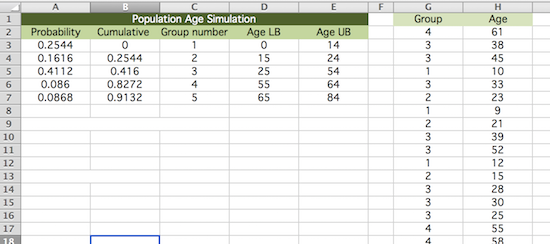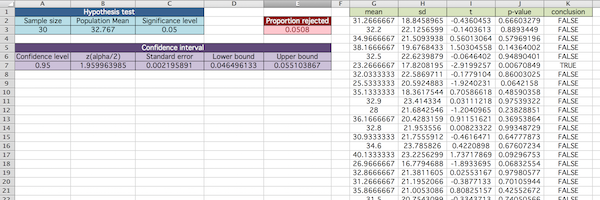Excel Project: Assumptions Matter!

The purpose of this task is to study an example where the assumptions of a hypothesis test are not met. In particular, we wish to see how this affects the probability of a type I error.

First, let us simulate a population of people's ages. According to the CIA World Factbook, the proportion of people in the world in the following age bands are as given below:

 14 years old or younger 25.44% 15 to 24 years old 16.16% 25 to 54 years old 41.12% 55 to 64 years old 8.6% 65 to 84 years old 8.68%

For simplicity, let's assume that we will not simulate anyone older than 84 years old.

Put this information into chart form, as shown below (with a green header), and then use it to generate an "age" population in the following way: select a random age band "group" in accordance with the probabilities just given, and then pick a uniformly random age in this age band. For convenience, you can list the group chosen in the column just left of the column corresponding to the ages chosen. (Remember the VLOOKUP() and INDEX() functions, they may be helpful!)Now, add another sheet and randomly sample from the "Age" column to make a table that is 50 columns by 10,000 rows. (Use F5 to select this large array. Control D and Control R will fill a formula down and to the right, respectively.) This represents 10,000 samples of size up to 50.

Our intention is - for EACH of these samples - to test whether they suggest there is evidence that the population mean is not what it actually is. To help in the calculations needed for all of these tests, let us create cells (colored blue below) in a third sheet that contain the desired sample size (30), the significance level (0.05) for our tests, and to calculate the population mean.

Find the mean and standard deviation of each of the 10,000 samples using the sample size entered. Then calculate each of the t-test statistics, the P-values, and decide whether to reject the null hypothesis. (Remember to reference the blue cells, as you make your calculations.)

In another cell (colored red below), find the proportion of samples for which the null hypothesis is rejected.1. For what proportion of samples did you expect the null hypothesis to be rejected? Carefully explain why.